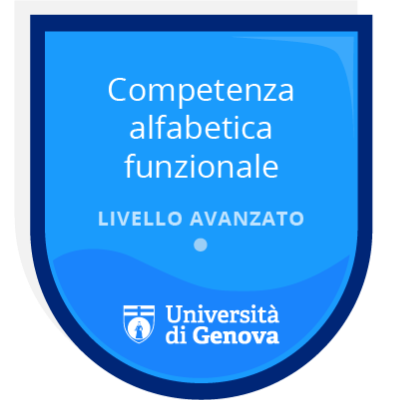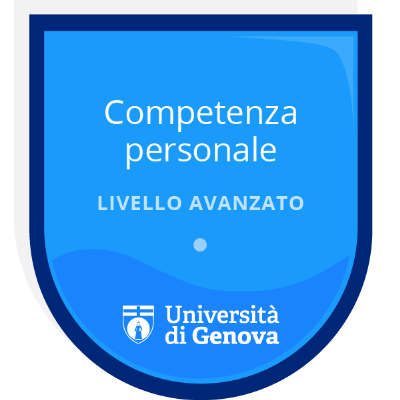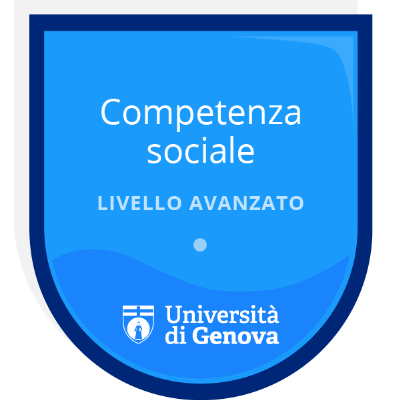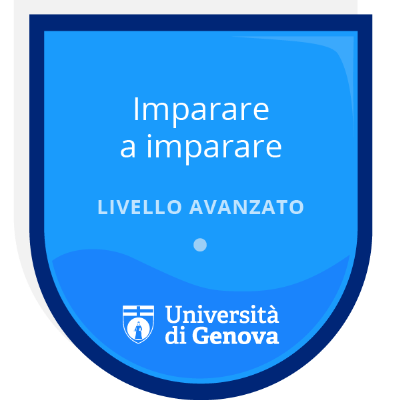CODE 108854 2022/2023 9 cfu anno 1 MARITIME SCIENCE AND TECHNOLOGY 10948 (L-28) - GENOVA FIS/01 GENOVA 1° Semester AULAWEB

## OVERVIEW

The Physics course deals with the fundamental laws of mechanics, hydromechanics, gravitation, waves and electricity essential for understanding any scientific and technological application the student will deal with during his training

## AIMS AND CONTENT

### LEARNING OUTCOMES

Understand basic physical fundamentals and the key vocabulary to describe them: kinematics, dynamics, work and energy, rotations, equilibrium, elasticity, gravitation, fluids, electricity, oscillations, and waves. Develop skills in observation, interpretation, reasoning, synthesis, generalizing, predicting, and questioning as a way to learn new knowledge. Develop scientific problem-solving skills, including organization of given information, identification and application of pertinent principles, quantitative solutions, interpreting results, and evaluating the validity of results. Apply conceptual understanding of the physics to general real-world situations and recognize how and when physics methods and principles can help address problems in their future courses and then apply those methods and principles to solve new problems.

### AIMS AND LEARNING OUTCOMES

Attendance and active participation in the proposed educational activities (lectures and exercises) and individual study will allow the student to:

- acquire the ability to describe and analyze the basic concepts concerning measurement of physical quantities

- understand and apply the fundamental laws of Kinematics

- understand and apply the fundamental laws of Dynamics to a variety of common situations

- understand, describe and apply the basic concepts of Kinetic and Potential Energy, Work and Power

- extend the fundamental laws of Kinetics and Dynamics to Rotation

- understand and apply the concepts of Equilibrium and Elasticity

- understand Gravitation laws and apply them to the motion of Earth and Satellites

- acquire the ability to describe and analyze the basic concepts regarding Fluid Statics and Dynamics applying them to real-life situations

- acquire understanding concerning common Oscillations modes and Mechanical Waves

- acquire a fundamental knowledge of Electricity and Electrical Circuits

The student will be encouraged to understand the advantages and limitations connected to the use of schematics and models, employ scientific language and formalism correctly, recognize the applicability of schematizations and models in real-life situations, set and solve exercises and problems within the proposed contents and critically evaluate the results.

### PREREQUISITES

Algebra and planar trigonometry

### TEACHING METHODS

The course is divided into several phases combining personal work by the student, group work and lectures given by the lecturer. The theoretical part will be mainly examined by the students through written material assigned by the teacher in view of the following lesson. Students are asked to comment on the material through an appropriate computer platform. During the frontal lesson, after the teacher has recalled the fundamental concepts previously learned by the students, highlighting the critical points, practical examples and problems will be shared with the students. In a first phase, students will have to tackle the problems individually, in order to acquire the ability to apply the concepts just recalled. In a second phase, group work is planned in which small groups of students compare their approach to the problem and continue their work until a solution is found. A third phase consists of one of the groups of students presenting the procedure used to solve the problem to the whole class. During these stages, the teacher's role is to guide students to the correct approach to the problem and the appropriate use of models of the appropriate physical laws.

Working students and students with certified SLD (Specific Learning Disorders), disability or other special educational needs are advised to contact the teacher at the beginning of the course to agree on teaching and examination arrangements so to take into account individual learning patterns, while respecting the teaching objectives.

### SYLLABUS/CONTENT

Measurement

Physical quantities, Standards, and Units, Precision and Significant Figures, Dimensional Analysis

Motion in one Dimension

Position, Displacement and Average Velocity, Instantaneous Velocity and Speed, Acceleration, Constant Acceleration, Free-fall Acceleration

Vectors

Vectors and their Components, Unit Vectors, Adding Vectors by Components, Multiplying Vectors

Motion in Two and Three Dimensions

Position and Displacement, Average Velocity and Instantaneous Velocity, Average Acceleration and Instantaneous Velocity, Projectile Motion, Uniform Circular Motion, Relative Motion in One Dimension, Relative Motion in Two Dimensions

Force and Motion

Newton’s First and Second Laws, Some Particular Forces, Applying Newton’s Laws, Frictional Forces, The Drag Force and Terminal Speed, The Dynamics of Uniform Circular Motion

Kinetic Energy and Work

Kinetic Energy, Work and Kinetic Energy: Energy Theorem, Work Done by a Constant Force, Work Done by a Variable Force, Power

Potential Energy and Conservation of Energy

Potential Energy, Conservation of Mechanical Energy, Work Done on a System by an External Force, Conservation of Energy

Center of Mass and Linear Momentum

Center of Mass, Newton’s Second Law for a System of Particles, Linear Momentum, Collision and Impulse, Conservation of Linear Momentum, Momentum and Kinetic Energy in Collisions, Elastic Collisions in One Dimension, Collisions in Two Dimensions, Systems with Varying Mass

Rotation

Rotational Variables, Rotation with Constant Angular Acceleration, Relating the Linear and Angular Variables, Kinetic Energyof Rotation, Calculating the Rotational Inertia, Torque, Newton’s Second Law for Rotation, Work and Rotational Kinetic Energy

Rolling, Torque, and Angular Momentum

Rolling as Translation and Rotation Combined, The Kinetic Energy of Rolling, The Forces of Rolling, Angular Momentum,Newton’s Second Law in Angular Form, The Angular Momentum of a System of Particles, The Angular Momentum of a RigidBody Rotating About a Fixed Axis, Conservation of Angular Momentum, Precession of a Gyroscope

Equilibrium and Elasticity

Equilibrium, The Center of Gravity, Some Examples of Static Equilibrium, Elasticity

Gravitation

Newton’s Law of Gravitation, Gravitation and the Principle of Superposition, Gravitation Near Earth’s Surface, Gravitational Potential Energy, Planets and Satellites: Kepler’s Laws, Satellites: Orbits and Energy

Fluid Statics

Fluids and Solids, Density and Pressure, Measurement of Pressure, Variations of Pressure in a Fluid at Rest, Pascal’s Principleand Archimedes’ Principle

Fluid Dynamics

General Concepts of Fluid Flow, Streamlines and the Equation of Continuity, Bernoulli’s Equation, Fields of Flow, Viscosity,Turbulence, and Chaotic Flow

Oscillations

Simple Harmonic Motion, The Force Law for Simple Harmonic Motion, Energy in Simple Harmonic Motion, Pendulums, Damped Simple Harmonic Motion, Forced Oscillations and Resonance

Waves

Transverse and Longitudinal Waves, Wave Speed on a Stretched String, Energy and Power of a Wave Traveling Along a String, The Wave Equation, The Principle of Superposition for Waves, Interference of Waves, Standing Waves and Resonance, Sound Waves, Beats, The Doppler Effect, Supersonic Speeds, Shock Waves

Coulomb’s Law and Electric Fields

Electric Charge, Conductors and Insulators, Coulomb’s Law, The Electric Field, The Electric Field Due to a Point Charge and to a Dipole, The Electric Field Due to a Line of Charge, The Electric Field Due to a Charged Disk, A Point Charge in an Electric Field, A Dipole in an Electric Field

Electric Potential

Equipotential Surfaces, Potential Due to a Charged Particle – to an electric Dipole – to a Continuous Charge Distribution, Electric Potential Energy of a System of Charged Particles, Potential of a Charged Isolated Conductor

Capacitance, Current, Resistance and Circuits

Capacitance, Capacitors in Parallel and in Series, Energy Stored in an Electric Field, Capacitor with a Dielectric, Electric Current, Current Density, Resistance and Resistivity, Ohm’s Law, Power in Electric Circuits, Work, Energy, and Emf, Calculating the Current in a Single-Loop Circuit, Potential Difference Between Two Points, Multiloop Circuits, RC Circuits.

Various texts cover the course material. A reference textbook for the Course is:

D. Halliday, R. Resnick, J. Walker, Fundamental of Physics: Extended, John Wiley & Sons

## TEACHERS AND EXAM BOARD

### Exam Board

DAVIDE FRANCESCO RICCI (President)

LORENZO PAPA

EUGENIA TORELLO (President Substitute)

## LESSONS

### LESSONS START

https://corsi.unige.it/10948/p/studenti-orario

### Class schedule

L'orario di tutti gli insegnamenti è consultabile all'indirizzo EasyAcademy.

## EXAMS

### EXAM DESCRIPTION

Written and oral exams.

Written exam

The examination consists of solving a few (typically 5) physics problems covering the main topics of the course.

Admission to the oral part of the exam only if the mark of the written exam is ≥ 16/30

Available only on official dates.

The student is allowed to repeat the complete test, but the previous result will then be cancelled

As an alternative to the written examination at the end of the semester, three partial tests will be carried out during the semester: the grade for the first and second test must be ≥ 12/30 to allow access to the last partial test, otherwise the student will pass directly to take the full written examination at the end of the semester. To take the oral exam, the average score for the three tests must be ≥ 15/30.

Oral examination

This consists of the presentation of a topic from the program of the course chosen by the board, followed by the student's analysis and solution of a written problem proposed by the board. Those who enter the oral examination having passed the three intermediate written tests may choose the topic of the first oral question as they wish.

### ASSESSMENT METHODS

The written examination will test the acquisition of basic knowledge of the main physical laws governing the mechanics of solids, the mechanics of liquids, gravitation, waves and electricity, as well as the ability to apply this knowledge to the solution of problems representative of practical situations. The student must be able to provide solutions to problems using a systematic approach by means of diagrams and models using the formal tools acquired during the course. The quality of exposition, the correct use of symbology and the accuracy of all formal steps used to arrive at the solution will be assessed.

The oral examination will test the acquisition of the ability to associate physical laws with each other and the ability to use them to provide a quantitative description of phenomena drawn from practical situations.

### Exam schedule

Data Ora Luogo Degree type Note
12/01/2023 14:30 GENOVA Scritto + Orale
09/02/2023 14:30 GENOVA Scritto + Orale
30/05/2023 14:30 GENOVA Scritto + Orale
15/06/2023 14:30 GENOVA Scritto + Orale
29/06/2023 14:30 GENOVA Scritto + Orale
19/07/2023 14:30 GENOVA Scritto + Orale

### FURTHER INFORMATION